SLASEL1D June   2017  – August 2018

PRODUCTION DATA.

1. Features
2. Applications
3. Description
1.     Device Images
4. Revision History
5. Device Comparison Table
6. Pin Configuration and Functions
7. Specifications
8. Detailed Description
1. 8.1 Overview
2. 8.2 Functional Block Diagram
3. 8.3 Feature Description
1. 8.3.1 Digital-to-Analog Converter (DAC) Architecture
2. 8.3.2 Internal Reference
3. 8.3.3 Device Reset Options
4. 8.4 Device Functional Modes
5. 8.5 Programming
6. 8.6 Register Map
1. 8.6.1 NOP Register (address = 0x00) [reset = 0x0000]
2. 8.6.2 DEVICE ID Register (address = 0x01) [reset = 0x---]
3. 8.6.3 SYNC Register (address = 0x2) [reset = 0xFF00]
4. 8.6.4 CONFIG Register (address = 0x3) [reset = 0x0000]
5. 8.6.5 GAIN Register (address = 0x04) [reset = 0x---]
6. 8.6.6 TRIGGER Register (address = 0x05) [reset = 0x0000]
7. 8.6.7 BRDCAST Register (address = 0x6) [reset = 0x0000]
8. 8.6.8 STATUS Register (address = 0x7) [reset = 0x0000]
9. 8.6.9 DACx Register (address = 0x8 to 0xF) [reset = 0x0000 or 0x8000]
9. Application and Implementation
1. 9.1 Application Information
2. 9.2 Typical Application
10. 10Power Supply Recommendations
11. 11Layout
12. 12Device and Documentation Support
13. 13Mechanical, Packaging, and Orderable Information

• RTE|16
• YZF|16
• RTE|16

### 7.6 Typical Characteristics

At TA = 25°C, VDD = 5.5 V, Internal Reference = 2.5 V, Gain = 2, DAC outputs unloaded, unless otherwise noted.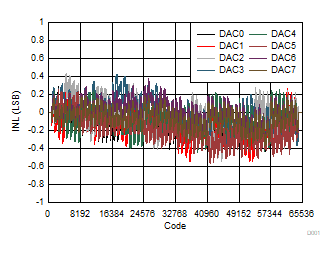Figure 1. Integral Linearity Error vs Digital Input Code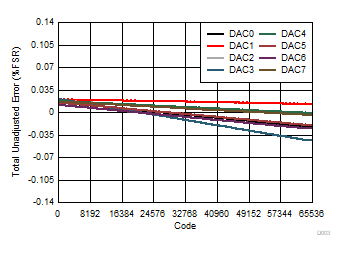Figure 3. Total Unadjusted Error vs Digital Input Code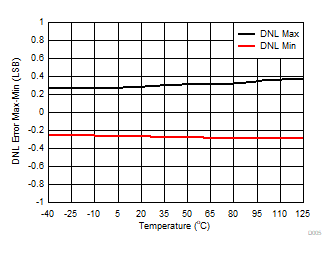Figure 5. Differential Linearity Error vs TemperatureFigure 7. Offset Error vs Temperature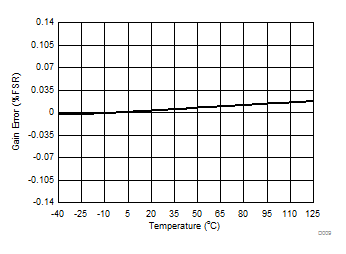Figure 9. Gain Error vs Temperature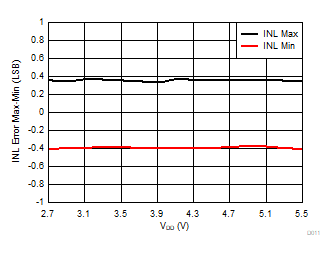Gain = 1
Figure 11. Integral Linearity Error vs Supply Voltage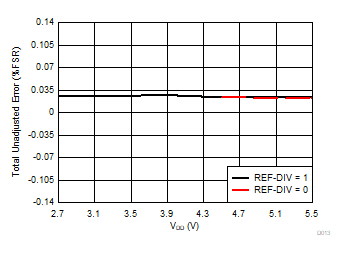Gain = 1
Figure 13. Total Unadjusted Error vs Supply Voltage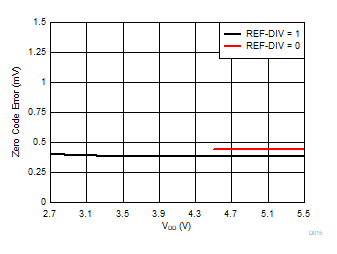Gain = 1
Figure 15. Zero Code Error vs Supply Voltage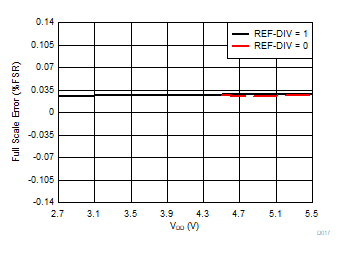Gain = 1
Figure 17. Full Scale Error vs Supply VoltageGain = 1
Figure 19. Differential Linearity Error vs Reference Voltage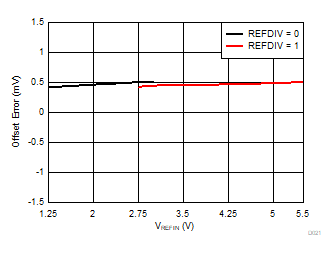Gain = 1
Figure 21. Offset Error vs Reference Voltage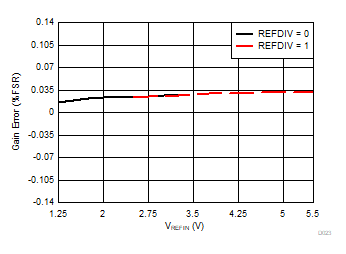Gain = 1
Figure 23. Gain Error vs Reference VoltageGain = 1. External Reference = 2.5 V
Figure 25. Supply Current with External Reference vs
Digital Input Code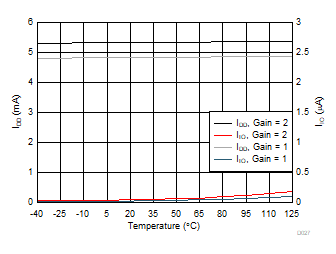Gain = 1. External Reference = 2.5 V
Figure 27. Supply Current with External Reference vs
Temperature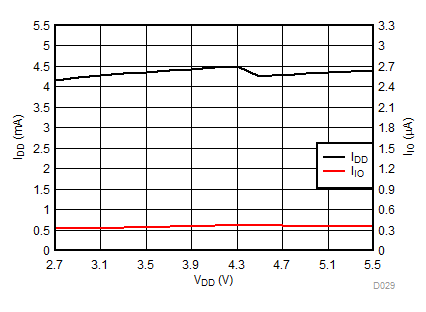Gain = 1. External Reference = 2.5 V
Figure 29. Supply Current with External Reference vs
Supply Voltage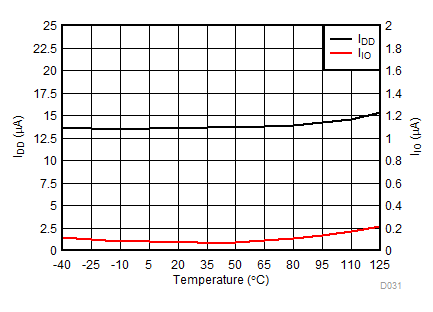Figure 31. Power Down Current vs Temperature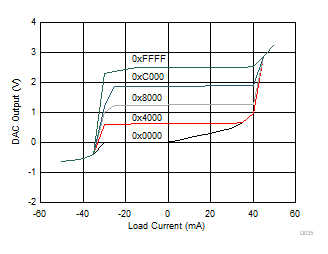Figure 35. Source and Sink Capability with Gain = 1Gain = 1
Figure 37. Full-Scale Settling Time, Rising EdgeGain = 1
Figure 39. Glitch Impulse, Falling Edge, 1 LSB Step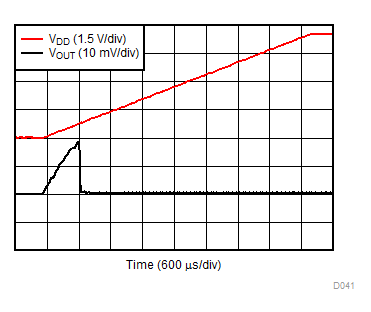Gain = 1
Figure 41. Power-On, Reset to Zero ScaleGain = 1
Figure 43. DACx0508C, Clear to Zero ScaleGain = 1. DAC code at midscale
Figure 45. VDD Power-Down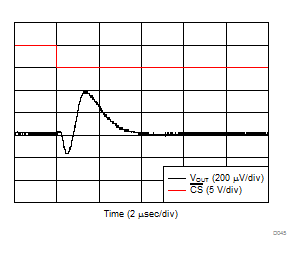Gain = 1. Measured DAC at midscale. All other DACs switch from code 32 to full scale
Figure 47. Channel to Channel CrosstalkGain = 1. VDD = 5 V + 200 mVPP (Sinusoid). DAC code at fullscale
Figure 49. DAC Output AC PSRR vs Frequency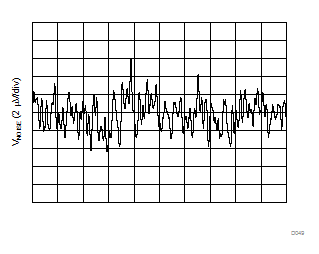Gain = 1. External Reference = 2.5 V. DAC code at midscale
Figure 51. DAC Output Noise with External Reference
0.1 Hz to 10 Hz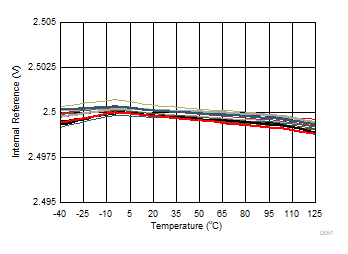Figure 53. Internal Reference Voltage vs TemperatureFigure 55. Internal Reference Voltage vs Time0.1 Hz to 10 Hz
Figure 57. Internal Reference Noise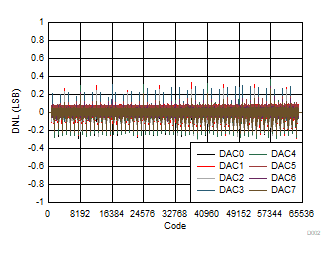Figure 2. Differential Linearity Error vs Digital Input Code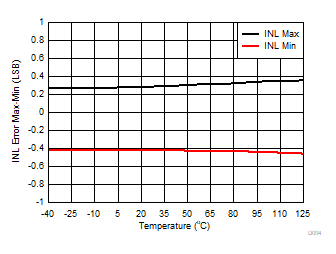Figure 4. Integral Linearity Error vs Temperature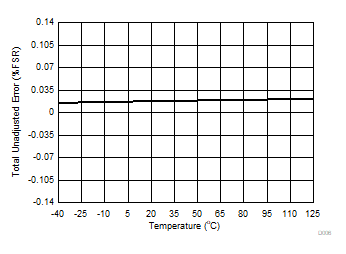Figure 6. Total Unadjusted Error vs Temperature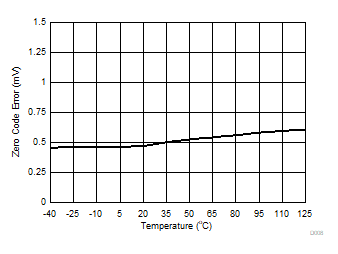Figure 8. Zero Code Error vs TemperatureFigure 10. Full Scale Error vs Temperature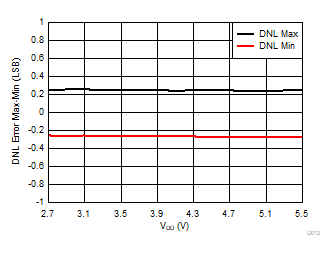Gain = 1
Figure 12. Differential Linearity Error vs Supply Voltage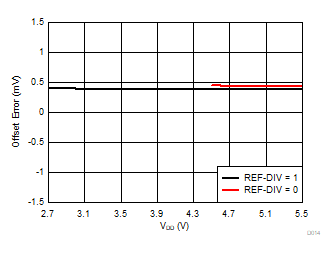Gain = 1
Figure 14. Offset Error vs Supply Voltage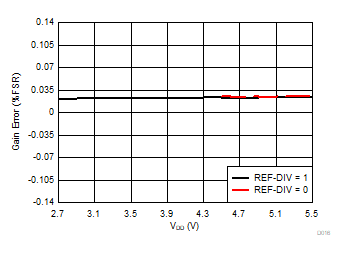Gain = 1
Figure 16. Gain Error vs Supply Voltage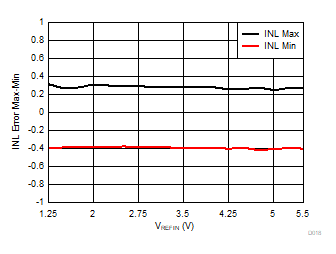Gain = 1
Figure 18. Integral Linearity Error vs Reference VoltageGain = 1
Figure 20. Total Unadjusted Error vs Reference Voltage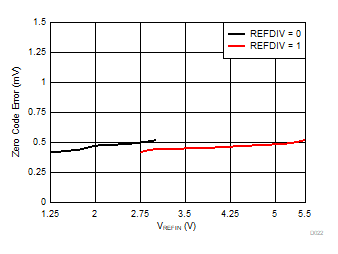Gain = 1
Figure 22. Zero Code Error vs Reference Voltage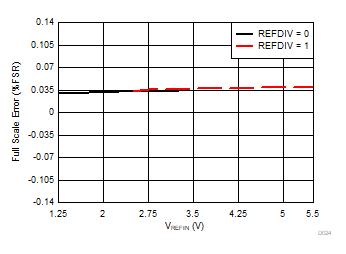Gain = 1
Figure 24. Full Scale Error vs Reference Voltage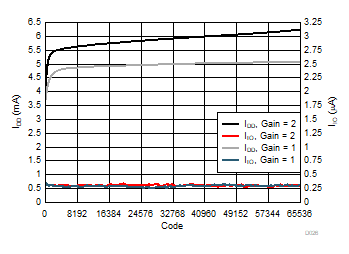Gain = 1
Figure 26. Supply Current with Internal Reference vs
Digital Input Code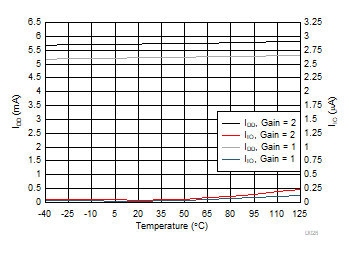Gain = 1
Figure 28. Supply Current with Internal Reference vs
Temperature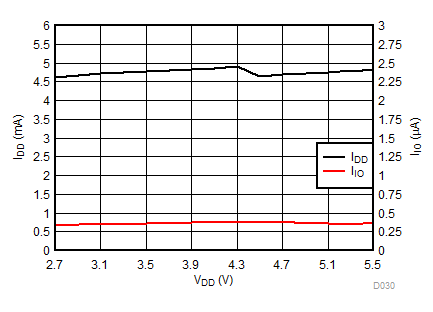Gain = 1
Figure 30. Supply Current with Internal Reference vs
Supply VoltageFigure 32. Power Down Current vs Supply VoltageFigure 34. Source and Sink Capability with Gain = ½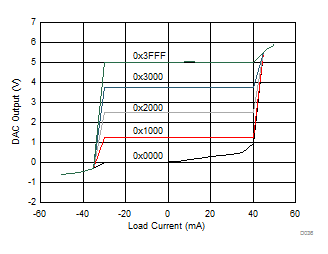Figure 36. Source and Sink Capability with Gain = 2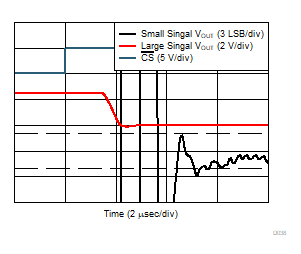Gain = 1
Figure 38. Full-Scale Settling Time, Falling Edge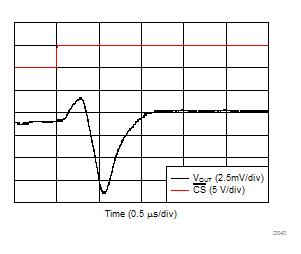Gain = 1
Figure 40. Glitch Impulse, Rising Edge, 1 LSB StepGain = 1
Figure 42. Power-On, Reset to Midscale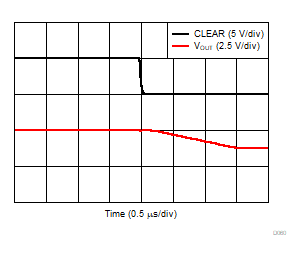Gain = 1
Figure 44. DACx0508C, Clear to Midscale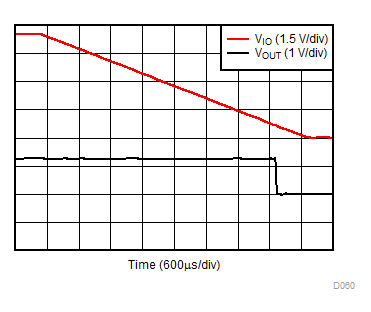Gain = 1. DAC code at midscale
Figure 46. VIO Power-DownGain = 1. DAC code at midscale
Figure 48. Clock Feedthrough with SCLK = 1 MHz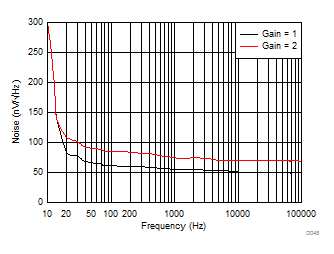External Reference = 2.5 V. DAC code at midscale
Figure 50. DAC Output Noise Density vs Frequency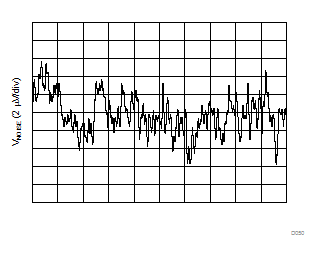Gain = 1. DAC code at midscale
Figure 52. DAC Output Noise with Internal Reference
0.1 Hz to 10 HzFigure 54. Internal Reference Voltage vs Supply Voltage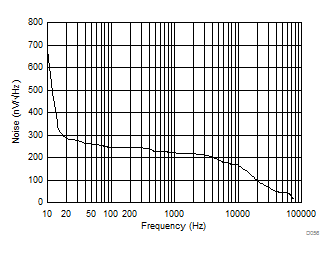Figure 56. Internal Reference Noise Density vs FrequencyFigure 58. Internal Reference Temperature Drift Histogram